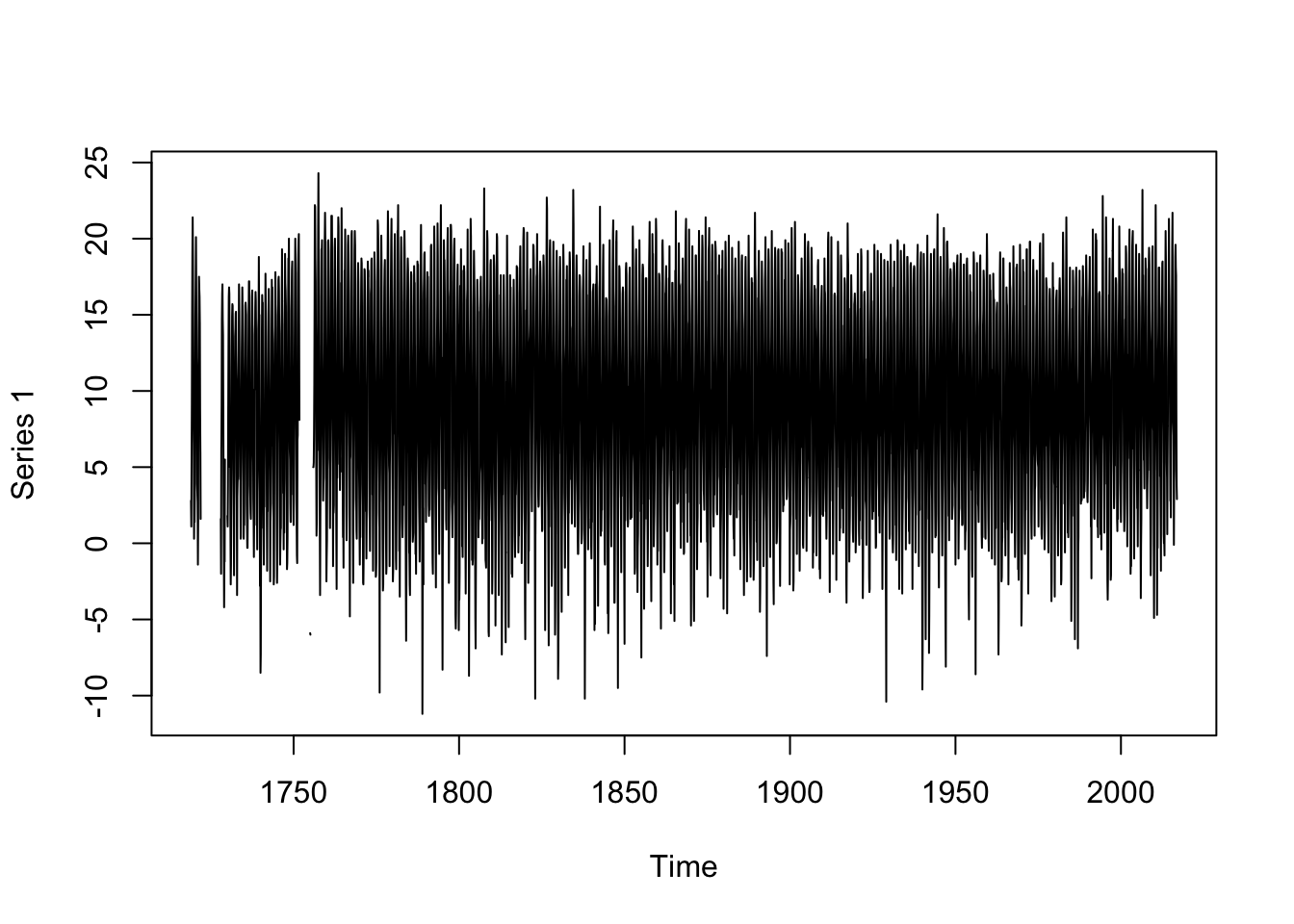In the following sections we introduce basic operations for time series analysis. We disuses the following topics:

• Subsetting and indexing
• Summary statistics
• Aggregation of time series data

As mentioned in the previous section there exist many different R-packages to work with time series. Please be aware that basic operations on time series are implemented in most of them. Hence, it is very important to be aware of the object class and respectively, the data representation. This representation dictates which functions will be available for loading, processing, analyzing, printing, and plotting the time series data.

For the purpose of demonstration we load the monthly (`ts.FUB.monthly`) and daily (`ts.FUB.daily`) time series data for the weather station Berlin-Dahlem (FU) into R. We can do that by using the `load()` function. Check out the previous section on Data sets used to remind yourself how we processed the data.

``````library(xts)

First, we check to object classes for the two data sets.

``class(ts.FUB.monthly)``
``##  "xts" "zoo"``
``class(ts.FUB.daily)``
``##  "xts" "zoo"``

Perfect, both data sets are of class `xts` and `zoo`. Note that `xts` is just an extension of the `zoo` class. Visit the vignettes for these both packages to refresh your knowledge on these type of data representation. Type `vignette('zoo')` and `vignette('xts')` into your console to open the vignettes.

For the sake of this tutorial we transform the monthly data set (`ts.FUB.monthly`) into a `ts` object, but keep the object type (`xts`) for the daily data set. Therefore, we apply the `ts()` function, which converts a vector into a time series. The `ts` object class is less flexible compared to the `zoo` or `xts` object classes, however, this object class is widely used and thus, it is important to know how to interact with that particular object class.

The `ts` function needs as least these arguments:

• `x`: a vector with data
• `start`: starting date of the series
• `end`: ending date of the series
• `frequency`: number of observations in the series per unit of time

In order to extract the vector with data (`x`) from the `xts` object, in our case the `ts.FUB.monthly` object, we apply the `coredata()` function on it.

``````x <- coredata(ts.FUB.monthly)
``````##      [,1]
## [1,]  2.8
## [2,]  1.1
## [3,]  5.2
## [4,]  9.0
## [5,] 15.1
## [6,] 19.0``````

Further, to extract the `start` argument we apply the `index()` function on of the `ts.FUB.monthly` object and combine it with the `year()` and `month()` function. The `start` argument is either a single number or a vector of two integers, which specify a natural time unit.

``````library(lubridate)
start <- c(year(index(ts.FUB.monthly)),
month(index(ts.FUB.monthly)))
start      ``````
``##  1719    1``

As we are dealing with monthly data we set the argument `frequency = 12`.

Putting it all together gives

``````ts.FUB.monthly = ts(x,
start = start,
frequency = 12)
str(ts.FUB.monthly)``````
``````##  Time-Series [1:3576, 1] from 1719 to 2017: 2.8 1.1 5.2 9 15.1 19 21.4 18.8 13.9 9 ...
##  - attr(*, "dimnames")=List of 2
##   ..\$ : NULL
##   ..\$ : chr "Series 1"``````

The `ts.FUB.monthly` contains a time series of monthly mean temperatures from January 1719 to December 2016.

Let us plot the `ts` object with the generic `plot()` function.

``plot(ts.FUB.monthly)``We could as well have used the `autoplot()` function from the `ggplot2` package. Let us now visualize the data set `ts.FUB.daily` using the `autoplot()` function. However, first we inspect the data set with the `str()` function.

``str(ts.FUB.daily)``
``````## An 'xts' object on 1950-01-01/2016-12-31 containing:
##   Data: num [1:24472, 1:2] -3.2 1 2.8 -0.1 -2.8 2.6 5.7 7 5.8 -2.4 ...
##  - attr(*, "dimnames")=List of 2
##   ..\$ : NULL
##   ..\$ : chr [1:2] "Temp" "Rain"
##   Indexed by objects of class: [Date] TZ: UTC
##   xts Attributes:
##  NULL``````

We see that the `ts.FUB.daily` time series ranges from 1950-01-01 to 2016-12-31. Further, we realize that the `xts` object `ts.FUB.daily` stores two time series: one for temperatures (`Temp`) and one for rainfall (`Rain`).

``````library(ggplot2)
autoplot(ts.FUB.daily)``````